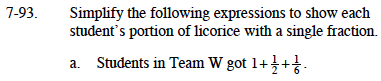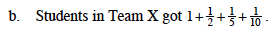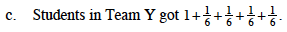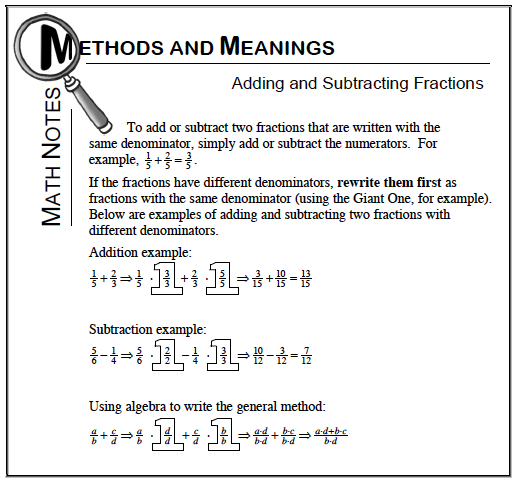### Home > MC1 > Chapter 7 > Lesson 7.2.5 > Problem7-93

7-93.Refer to the Math Notes box below for adding fractions.What denominator could you choose for this problem?Remember it should be a multiple of each of the denominators.

$1\frac{2}{3}$

$1\frac{4}{5}$Do you need to find a new denominator for this problem? Why or why not?